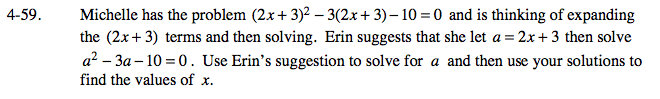### Home > PC > Chapter 4 > Lesson 4.1.4 > Problem4-59

4-59.

Michelle has the problem (2x + 3)2 − 3(2x + 3) − 10 = 0 and is thinking of expanding the (2x + 3) terms and then solving. Erin suggests that she let a = 2x + 3 then solve a2 − 3a − 10 = 0. Use Erin’s suggestion to solve for a and then use your solutions to find the values of x. Homework Help ✎Solve for the values of a first.
Then substitute the values of a into a = 2x + 3.
Finally, solve for x. You should get two answers.